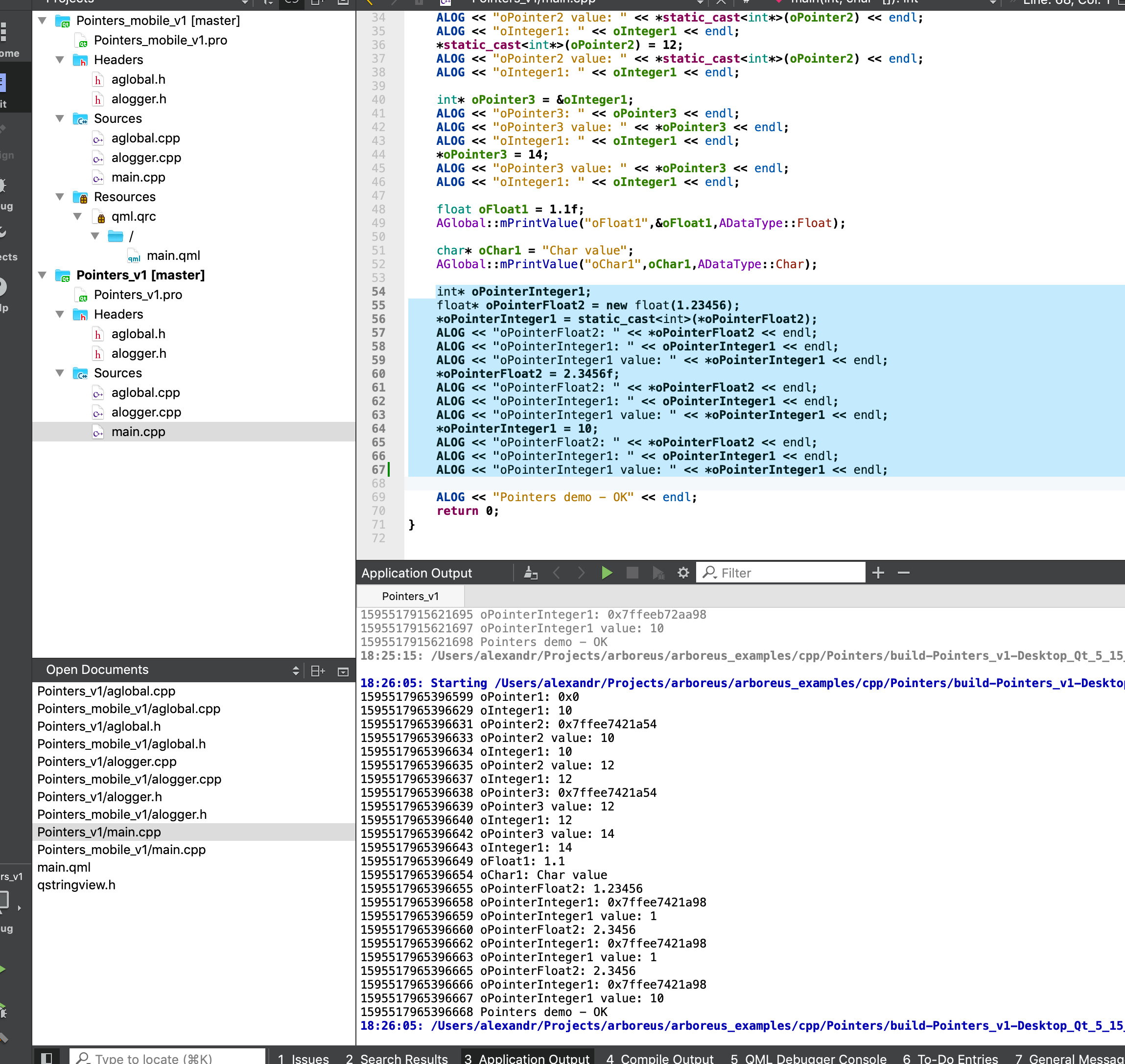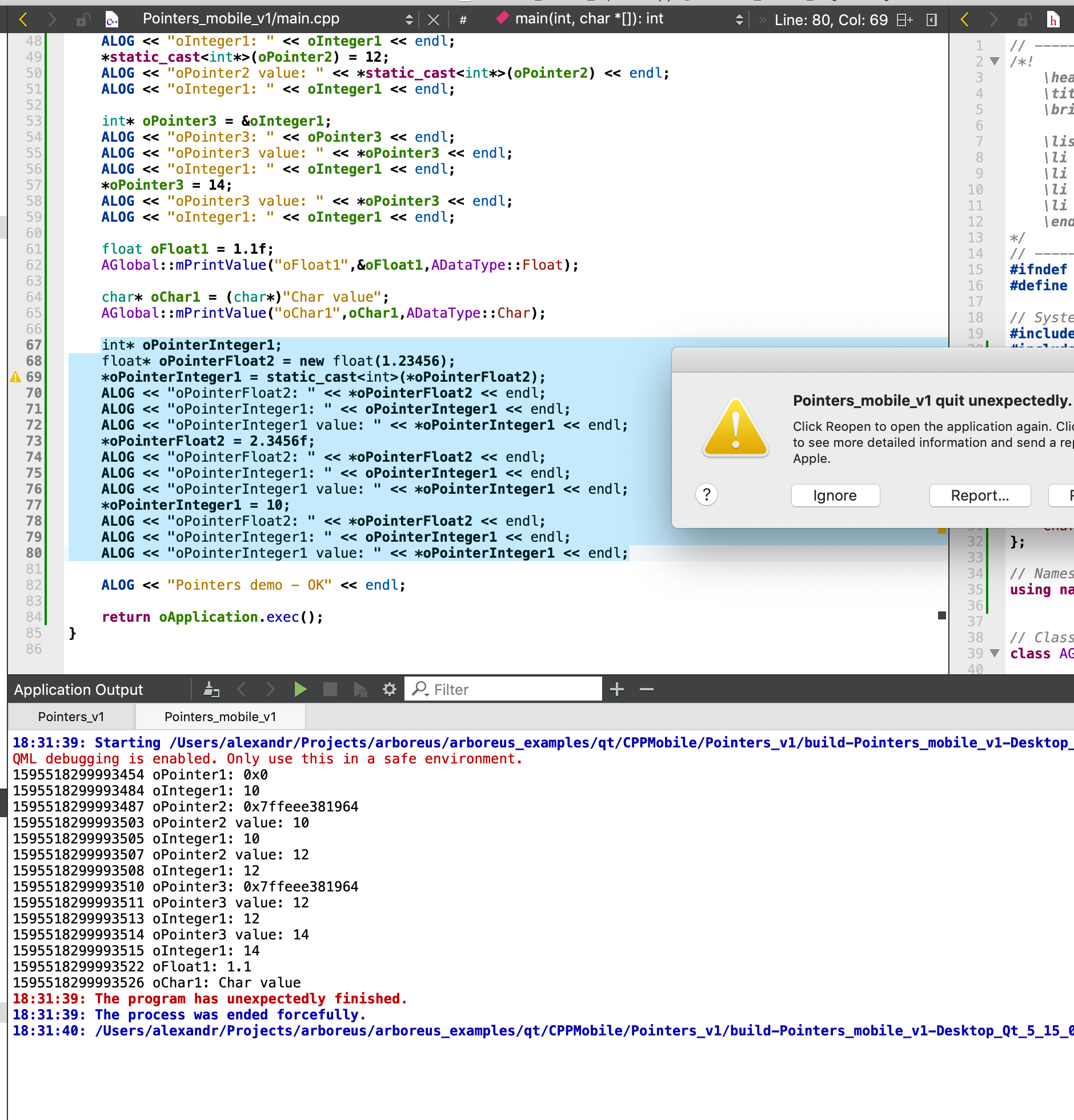# Qt Application crashed. Why?

• Hello!

I am just playing around CPP pointers (just for refreshing my memory). And got the issue when all is OK when it's plain CPP application (here the full code) and crashing when I am including it into QT/QML application (here the full code). This code:

``````	int* oPointerInteger1;
float* oPointerFloat2 = new float(1.23456);

*oPointerInteger1 = static_cast<int>(*oPointerFloat2);   << This line is crashing

ALOG << "oPointerFloat2: " << *oPointerFloat2 << endl;
ALOG << "oPointerInteger1: " << oPointerInteger1 << endl;
ALOG << "oPointerInteger1 value: " << *oPointerInteger1 << endl;
*oPointerFloat2 = 2.3456f;
ALOG << "oPointerFloat2: " << *oPointerFloat2 << endl;
ALOG << "oPointerInteger1: " << oPointerInteger1 << endl;
ALOG << "oPointerInteger1 value: " << *oPointerInteger1 << endl;

*oPointerInteger1 = 10;   << This line crashing too

ALOG << "oPointerFloat2: " << *oPointerFloat2 << endl;
ALOG << "oPointerInteger1: " << oPointerInteger1 << endl;
ALOG << "oPointerInteger1 value: " << *oPointerInteger1 << endl;
``````

The question is what is killing Qt/QML application? In this case it's working perfectly when it's plain CPP application. What am I missing?

All is working when I am changing this line:

``````int* oPointerInteger1;
``````

onto

``````int* oPointerInteger1 = new int(0);
``````

• *oPointerInteger1 = static_cast<int>(*oPointerFloat2); << This line is crashing

==> as far as I can see, you did not initialise `oPointerInteger1`, so you write on undefined address.
C/C++ pointers basics => https://www.tutorialspoint.com/cprogramming/c_pointers.htm

• @KroMignon But it's working perfectly on Plain CPP application. There are only trouble with Qt/QML application. There are published examples. The declaring and assigning - is the solution to avoid the crash? The only declaring - is there reason of Qt/QML crash. I am trying to get why is that only for Qt/QML and OK for plain CPP. From the point of view of syntax of pure CPP - all is OK. The question is about QT/QML.

• But it's working perfectly on Plain CPP application.

No this cannot work, uninitialized pointer == undefined behavior.

``````int* oPointerInteger1;
float* oPointerFloat2 = new float(1.23456);

*oPointerInteger1 = static_cast<int>(*oPointerFloat2);

``````

This is correct:

``````int* oPointerInteger1;
float* oPointerFloat2 = new float(1.23456);

int myBeautifulVariable;

oPointerInteger1 = &myBeautifulVariable;
*oPointerInteger1 = static_cast<int>(*oPointerFloat2);

``````

• @KroMignon Just try it ... And see how it works ...It's working perfectly when plain CPP.
And crashing when it's inside of Qt/QML.• You are playing programming roulette. Eventually you will shoot yourself.

• Dude, that is exactly what `undefined behavior` means.
Not crash doesn't mean it is not wrong.

• Also, you didn't copy the "published" code correctly:

``````int* oPointerInteger1;
``````

Should be:

``````int* oPointerInteger1 = new int(0);
``````

• Hi

But it's working perfectly on Plain CPP application.

Actually, in a plain cpp app, there is less it can randomly hit that will cause a crash.
But with QML/Qt loaded, far more to damage by writing int to random memory location.

so its random and will also crash in a pure CPP app if it randomly hits that